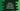# Python math.hypot method explanation with example## math.hypot() method in Python :

Defined in the math library, hypot() method is used to find the value of euclidean norm. For two numbers x and y, the euclidean norm is the length of the vector from origin (0,0) to the coordinate (x,y). For a point (x,y), it is equal to sqrt(xx + yy).

This method can be used to find out the hypotenuse of a right-angle triangle considering x and y as its two sides.

## Syntax of math.hypot() :

The syntax of math.hypot() function is as below :

``math.hypot(x,y)``

Both x and y should be numerical values. Otherwise, it will throw one error.

### Return value and error :

The return value of this method is of type float and it is the euclidean norm. It will throw one TypeError if we pass more than two arguments or if one of the arguments is of a different types.

### Example program :

Let me show you an example. This program will take two numbers as input from the user and print out the hypot value :

``````import math

x = int(input("Enter the first number : "))
y = int(input("Enter the second number : "))

print("math.hypot({},{}) : {}".format(x, y, math.hypot(x, y)))``````

### Sample outputs :

``````Enter the first number : 2
Enter the second number : 2
math.hypot(2,2) : 2.8284271247461903

Enter the first number : 3
Enter the second number : 3
math.hypot(3,3) : 4.242640687119285``````